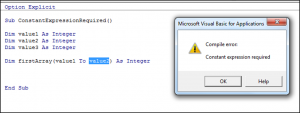# VBA Constant Expression Required

We covered arrays, static arrays and dynamic arrays in a previous tutorial.  We are going to look at a common error associated with static arrays called Constant Expression Required. This error is generated when you try to use a static array instead of a dynamic array as shown in the code below:The static array needs to have constants used to set it since it is fixed.
The way to resolve this error is to use a Dynamic array variable instead. You would use the ReDim keyword every time you want to resize the array. This is shown in the code below:

``````Sub UsingReDim()

Dim value1 As Integer
Dim value2 As Integer
Dim value3 As Integer

value1 = 3
value2 = 9
value3 = 15

Dim listofvalues() As Integer

ReDim listofvalues(value1)

End Sub``````

Stop searching for VBA code online. Learn more about AutoMacro - A VBA Code Builder that allows beginners to code procedures from scratch with minimal coding knowledge and with many time-saving features for all users!